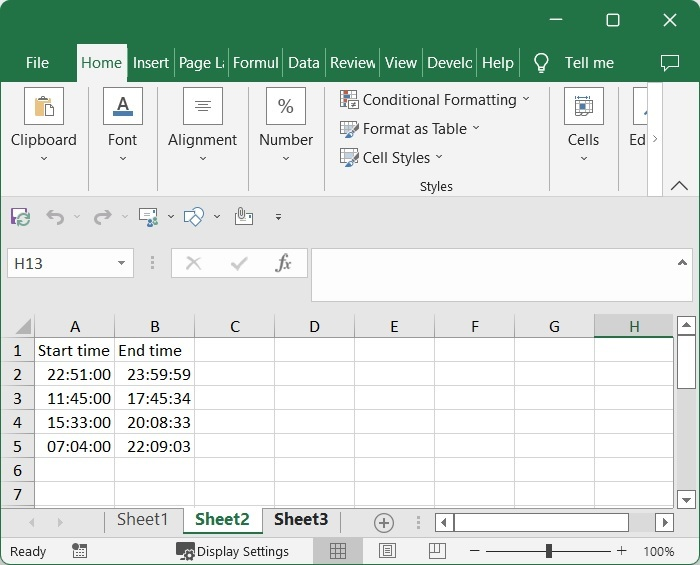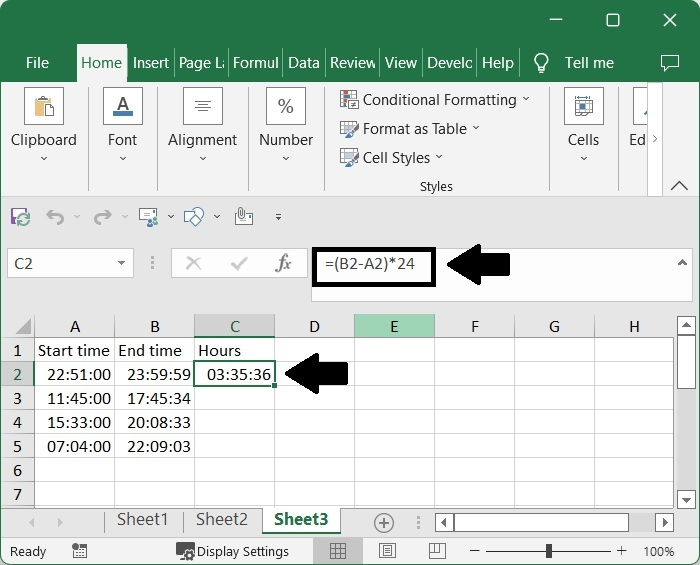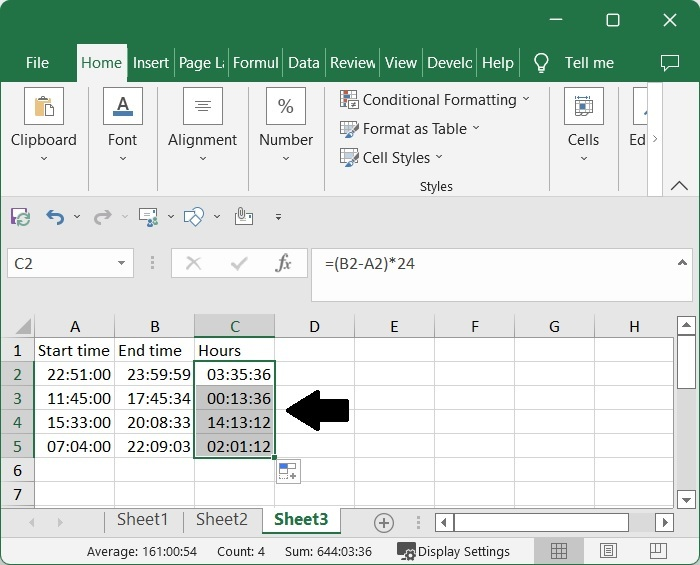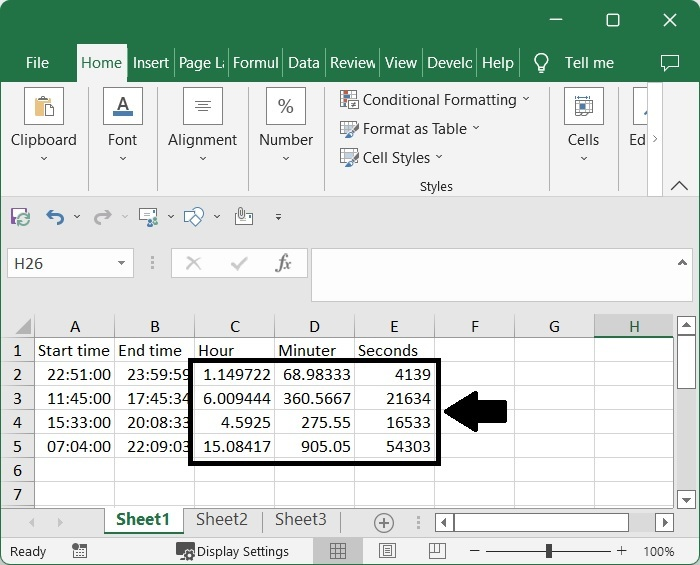# How to Convert Time Difference between Two Times to Number (Hours/Minutes/Seconds) in Excel?

In general, we will need to work with times more frequently in Excel. If we try to perform the difference between two different times manually, then it can be a complex and time-consuming process. When we calculate the time difference between two different times directly using the general formula, the value will be returned in hours, minutes, and seconds.

Sometimes you need the difference only in hours, minutes, or seconds. We can use the process discussed in this tutorial to complete this task. Read this tutorial to learn how you can convert the time difference between two times to only return a solution in hours, minutes, or seconds.

## Converting Time Difference between Two Times to Hours in Excel

Here we will first convert any one result into a number format using the formula, then get all the results using the auto-fill handle. Let us see a simple process to know how we can convert the time difference between two times to return the solution as a number of hours in Excel.

### Step 1

Let us consider an Excel sheet where the data is a list of start and end times, similar to the below image.Now click on an empty cell, in our case cell C2, and enter the formula as =(B2-A2)*24 and click enter to get our first result, as shown in the below image. In the formula, A2 and B2 are the addresses of start and end times, respectively, on the excel sheet.

Empty cell > Formula > Enter### Step 2

Then drag down from the first result using the auto-fill handle, as our final result will be similar to the below image.### Step 3

If the solutions returned are still in time format. Select the data, right-click, and select format cells to open a pop-up window. In the pop-up window, click on "General" and then "OK."

Select result > Right click > Format cells > General > OKIf we need the solution in minutes, we can use the formula =(B2-A2)*1440, and for seconds, we can use =(B2-A2)*86400.

## Conclusion

In this tutorial, we used a simple example to demonstrate how you can convert time differences into numbers in Excel.